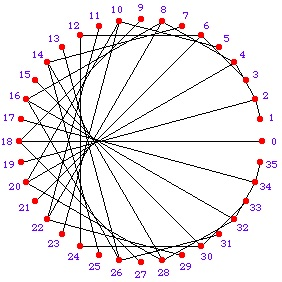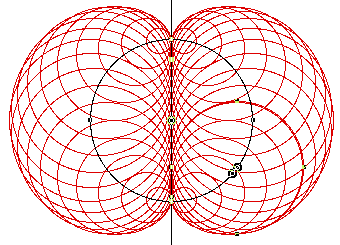Cardioid technology is used in microphones and speakers. Cardioids appear in the motion of planets and in many other ways. See this YouTube video.

To draw a cardioid first draw a circle and carefully mark 36 points around the circle at 10 degree intervals.

Join the points with straight lines as in the diagram. Number the points. Double each number and join it to the point given by the remainder after dividing by 36 (clock arithmetic).

For example 19 is joined to 2 because 2 times 19 is 38 which is 1 x 36 + 2. Similarly 25 is joined to 14 because 2 times 25 is 50 which is1 x 36 + 14.

This shape is called a nephroid. Start with a base circle and a diameter of that circle. Construct the nephroid by drawing circles with centres on the base circle and each tangent to the diameter of the base circle.

The cardioid and nephroid are examples of envelopes which are curves that are tangent at each point of contact to a family of curves.

If you join the positions of pairs of planets in our Solar System at different times you get these patterns. This happens because the motion of the planets around the sun is periodic. The video (link above) shows the pattern for the Earth and Venus.

Tagged with:

Set your Twitter account name in your settings to use the TwitterBar Section.